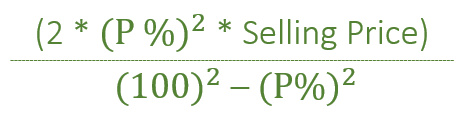# Loss when two items are sold at same price and same percentage profit/loss

• Difficulty Level : Basic
• Last Updated : 09 Jun, 2022

Given the Selling price i.e ‘SP’ of the two items each. One item is sold at ‘P%’ Profit and other at ‘P%’ Loss. The task is to find out the overall Loss.
Examples:

```Input: SP = 2400, P = 30%
Output: Loss = 474.725

Input: SP = 5000, P = 10%
Output: Loss = 101.01```

Approach:How does the above formula work?
For profit making item :
With selling price (100 + P), we get P profit.
With selling price SP, we get SP * (P/(100 + P)) profit
For loss making item :
With selling price (100 – P), we get P loss.
With selling price SP, we get SP * (P/(100 – P)) loss
Net Loss = Total Loss – Total Profit
= SP * (P/(100 – P)) – SP * (P/(100 + P))
= (SP * P * P * 2) / (100*100 – P*P)
Note: The above formula is applicable only when the Cost price of both the items are different. If CP of both the items are same then, in that case, there is ‘No profit No loss’.
Below is the implementation of the above approach

## C++

 `// C++ implementation of above approach.` `#include ` `using` `namespace` `std;`   `// Function that will` `// find loss` `void` `Loss(``int` `SP, ``int` `P)` `{`   `    ``float` `loss = 0;`   `    ``loss = (2 * P * P * SP) / ``float``(100 * 100 - P * P);`   `    ``cout << ``"Loss = "` `<< loss;` `}`   `// Driver Code` `int` `main()` `{` `    ``int` `SP = 2400, P = 30;`   `    ``// Calling Function` `    ``Loss(SP, P);`   `    ``return` `0;` `}`

## Java

 `// Java implementation of above approach.` `class` `GFG ` `{`   `// Function that will` `// find loss` `static` `void` `Loss(``int` `SP, ``int` `P)` `{`   `    ``float` `loss = ``0``;`   `    ``loss = (``float``)(``2` `* P * P * SP) / (``100` `* ``100` `- P * P);`   `    ``System.out.println(``"Loss = "` `+ loss);` `}`   `// Driver Code` `public` `static` `void` `main(String[] args) ` `{` `    ``int` `SP = ``2400``, P = ``30``;`   `    ``// Calling Function` `    ``Loss(SP, P);` `}` `}`   `// This code has been contributed by 29AjayKumar`

## Python3

 `# Python3 implementation of above approach. `   `# Function that will find loss ` `def` `Loss(SP, P): ` `    `  `    ``loss ``=` `0` `    ``loss ``=` `((``2` `*` `P ``*` `P ``*` `SP) ``/` `            ``(``100` `*` `100` `-` `P ``*` `P)) ` `    ``print``(``"Loss ="``, ``round``(loss, ``3``)) `   `# Driver Code ` `if` `__name__ ``=``=` `"__main__"``:`   `    ``SP, P ``=` `2400``, ``30`   `    ``# Calling Function ` `    ``Loss(SP, P) `   `# This code is contributed by Rituraj Jain`

## C#

 `// C# implementation of above approach.` `class` `GFG ` `{`   `// Function that will` `// find loss` `static` `void` `Loss(``int` `SP, ``int` `P)` `{`   `    ``double` `loss = 0;`   `    ``loss = (``double``)(2 * P * P * SP) / (100 * 100 - P * P);`   `    ``System.Console.WriteLine(``"Loss = "` `+ ` `                            ``System.Math.Round(loss,3));` `}`   `// Driver Code` `static` `void` `Main() ` `{` `    ``int` `SP = 2400, P = 30;`   `    ``// Calling Function` `    ``Loss(SP, P);` `}` `}`   `// This code has been contributed by mits`

## PHP

 ``

## Javascript

 ``

Output

`Loss = 474.725`

Time Complexity: O(1)
Auxiliary Space: O(1)

My Personal Notes arrow_drop_up
Recommended Articles
Page :